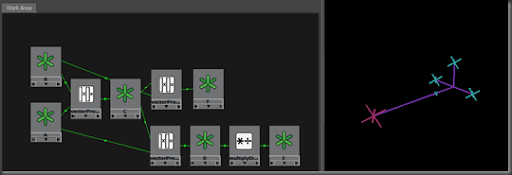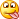## Saturday, February 2, 2013

### Understanding 3D Vectors

DISCLAIMER: This post covers the vector type in Maya. If you are new to vectors, please acquaint yourself with them and review the help docs before reading this post. I tried to keep this post as light on math as possible, however, I will clarify where needed, based on your suggestions. This is a huge topic and this post merely summarizes how useful vectors are.

User Guide > Scripting > MEL and Expressions > Useful functions

Let’s break one of our toys apart and learn how things work from the inside out. The knowledge we acquire from doing this allows us to build bigger and better toys. In physics, a vector is described as an entity that has both a magnitude and a direction. In MEL, a vector is represented by x, y, and z components. These components can be combined back into their scientific form at any time. Vectors can represent a location in space, the velocity and/or acceleration of an object, tracing rays between two points, etc.

Retrieving a vector’s magnitude is trivial in MEL:

vector \$A = << 1, 2, 3>>;
vector \$B = << 3, 2, 1>>;
int \$length = mag(\$A);

Finding the direction vector can by done by using the unit function.

When you perform arithmetic with vectors, the output is considered the resultant. Addition yields a vector that is said to be a diagonal in a parallelogram.

This is interesting to us because if you were to take the B and move a copy (by adding it to A) to the head of A, we build ourselves a triangle that can used to solve for solutions. If we then move a copy of A to the head of B, we end up with a parallelogram. When you subtract a vector from another, the resultant yields a magnitude that is the distance between the heads of A and B. If you take B-A (, which is not the same as A-B, ) and add it to A, we have ourselves another triangle. Still with me? This is only possible because a vector can be equal (& parallel) to any other vector in space as long as they are equal in direction and magnitude. So if you need to reposition a vector, do so by adding it to another vector location. That was the heaviest paragraph in this post, I promise!

By multiplying a vector by a scalar value, we are simply scaling the the vector’s magnitude by that number. There are other types of vector products that are extremely important in computer graphics, the dot product, which yields a scalar value, and the cross product (, which yields another vector perpendicular to the first two. Nuff talk, let’s get to work.

In Maya, create 4 locators. Name them A, B, C, & D. In the script editor, retrieve their world space positions and store those values in vectors. Imagine each locator to be a ray, that originates at the origin, and each locator shape being the vector’s head. Spread the locators in space away from the origin and each other, arbitrarily and for variety.

Make C perpendicular to the first two, by taking the cross product of A & B and move C to the resultant. Now that you know that C perpendicular to the first two, there’s a good chance there is one axis that is not perpendicular to another. You can verify this by taking the dot product of two vectors. If the dot product is not 0, then your vectors are not perpendicular.

TIP: Make the result of AxC a unit vector, a vector with the length 1, by using the unit function on C. The 'x' in this notation signifies we're taking the cross product of these two vectors.

Anyway, take the cross product of A & C, and feed the result to B. You now have 3 axis that are perfectly orthogonal to each other. Assuming that A was already at the “lookAt” location, let’s place D along A’s axis, but with a magnitude of 1. Vectors of this length are called unit vectors. The process of getting this result is call normalization.

Normalize A and pipe the results right into D. You now have solved for the same things that the aim constraint solves for in most 3D software, including games. If you were to normalize all 3 orthogonal axes, you would have what is called an Orthonormal Basis.

Here's a code snippet:

// 3D (Basis) Vectors
// By Lewis M., 2013
// Usage:

// Arbitrarily place four locators in the scene
// Name them A, B, C, & D

// Retrieve A & B's locations
float \$a_coord[] = `xform -q -ws -t A`;
float \$b_coord[] = `xform -q -ws -t B`;

// Find an orthogonal vector to both A & B

vector \$a = <<\$a_coord, \$a_coord, \$a_coord>>;
vector \$b = <<\$b_coord, \$b_coord, \$b_coord>>;
vector \$c = cross(\$a, \$b);
vector \$d;

// Scale it down to the length of 1
\$c = unit(\$c);
move -a (\$c.x) (\$c.y) (\$c.z) C;
print dot(\$a, \$b);
// Most likely not zero

// Adjust B to be orthogonal to both A and C
\$b = cross(\$a, \$c);
\$b = unit(\$b);
move -a (\$b.x) (\$b.y) (\$b.z) B;

// Finally, scale down A and place D in the position
\$d = unit(\$a);
move -a (\$d.x) (\$d.y) (\$d.z) D;

// Just for fun, draw some visually appealing curves
curve -d 1 -p 0 0 0 -p (\$a.x) (\$a.y) (\$a.z) -k 0 -k 1 ;
curve -d 1 -p 0 0 0 -p (\$b.x) (\$b.y) (\$b.z) -k 0 -k 1 ;
curve -d 1 -p 0 0 0 -p (\$c.x) (\$c.y) (\$c.z) -k 0 -k 1 ;

//grid -tgl 0;
//refresh;

As an exercise, you can use MEL, Python, or even utility nodes to create the connections.Above is a picture of my little aim constraint node setup.  It differs from my discussion above because this version is interactive. In this version, I am able to position A wherever I want and have the other locators automatically update. The NURBS curves you see there are strictly for visual appeal1.2.